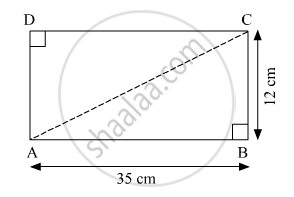Share

# Find the Diagonal of a Rectangle Whose Length is 35 Cm and Breadth is 12 Cm. - Geometry

ConceptPythagoras Theorem

#### Question

Find the diagonal of a rectangle whose length is 35 cm and breadth is 12 cm.

#### SolutionAccording to Pythagoras theorem, in ∆ABC

${AB}^2 + {BC}^2 = {AC}^2$
$\Rightarrow {35}^2 + {12}^2 = {AC}^2$
$\Rightarrow 1225 + 144 = {AC}^2$
$\Rightarrow {AC}^2 = 1369$
$\Rightarrow AC = 37 cm$

Hence, the length of the diagonal is 37 cm.

Is there an error in this question or solution?

#### APPEARS IN

Balbharati Solution for Balbharati Class 10 Mathematics 2 Geometry (2018 to Current)
Chapter 2: Pythagoras Theorem
Practice Set 2.1 | Q: 8 | Page no. 39

#### Video TutorialsVIEW ALL 

Solution Find the Diagonal of a Rectangle Whose Length is 35 Cm and Breadth is 12 Cm. Concept: Pythagoras Theorem.
S